## Review: Supervised and Unsupervised Learning

• So far we’be been talking about supervised learning. This is a problem where you have data $$X$$ and labels $$Y$$ and your goal is to learn a function that maps from the data $$X$$ $$\rightarrow$$ labels $$Y$$. Some examples of this are classification, regression, object detection etc.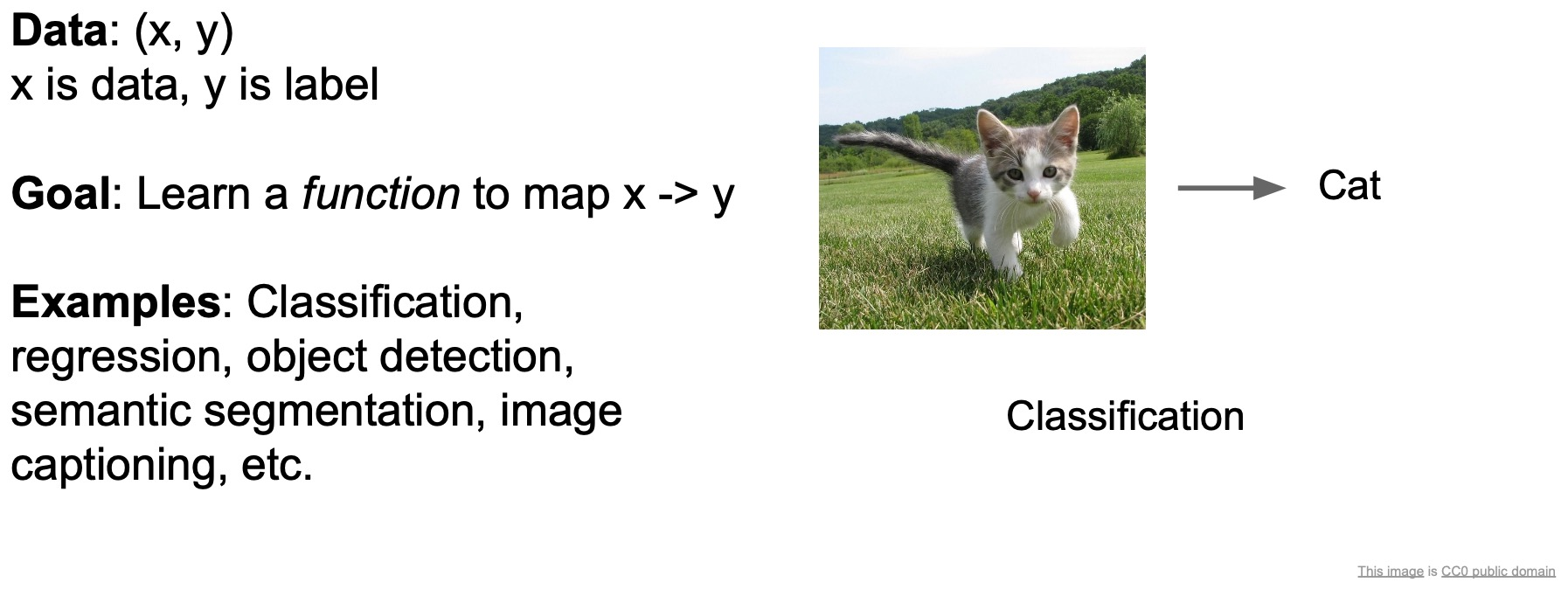• We’ve also talked about unsupervised learning where you only have the data and no labels. The goal here is to learn some underlying hidden structure in the data. For e.g., clustering, dimensonality reduction, density estimation, generative models are pretty good examples of this type of learning.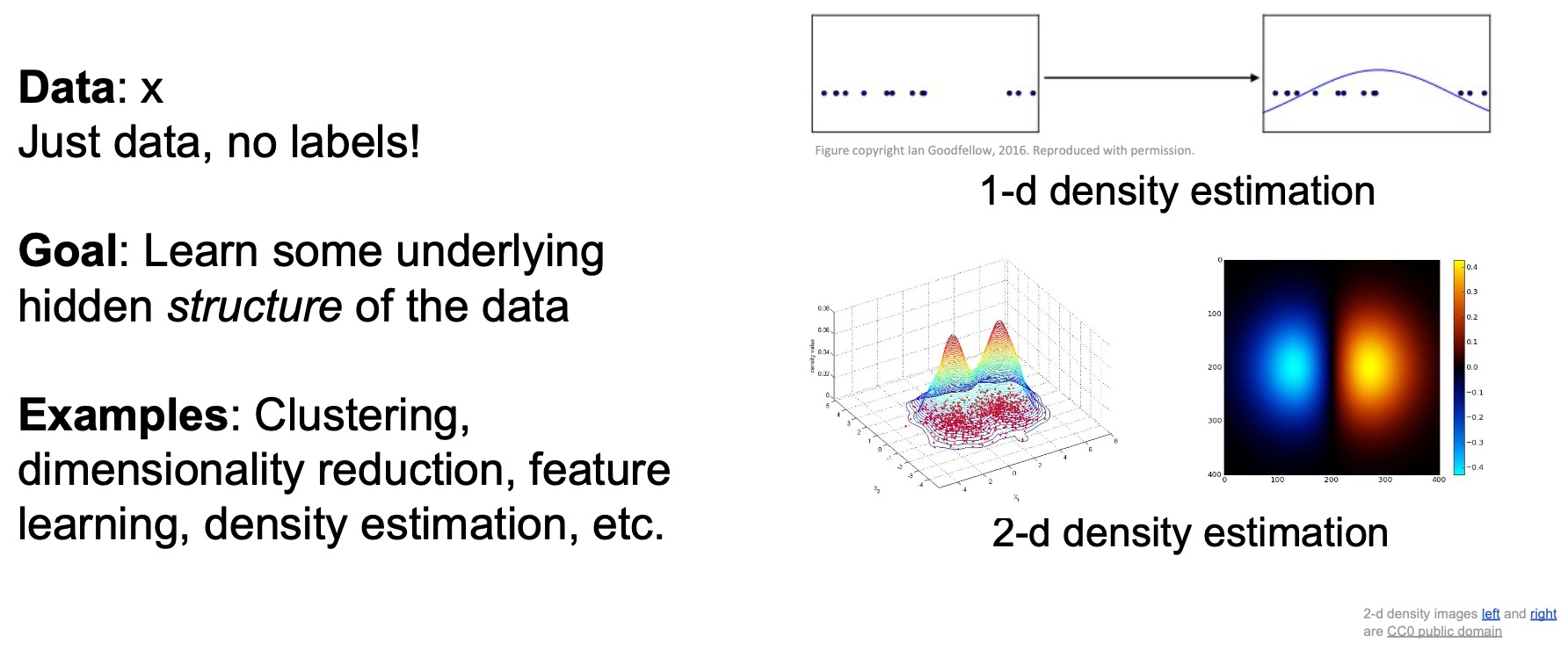• Today, we’re going to talk about a different type of problem – the reinforcement learning problem.

## Reinforcement Learnings: The Basics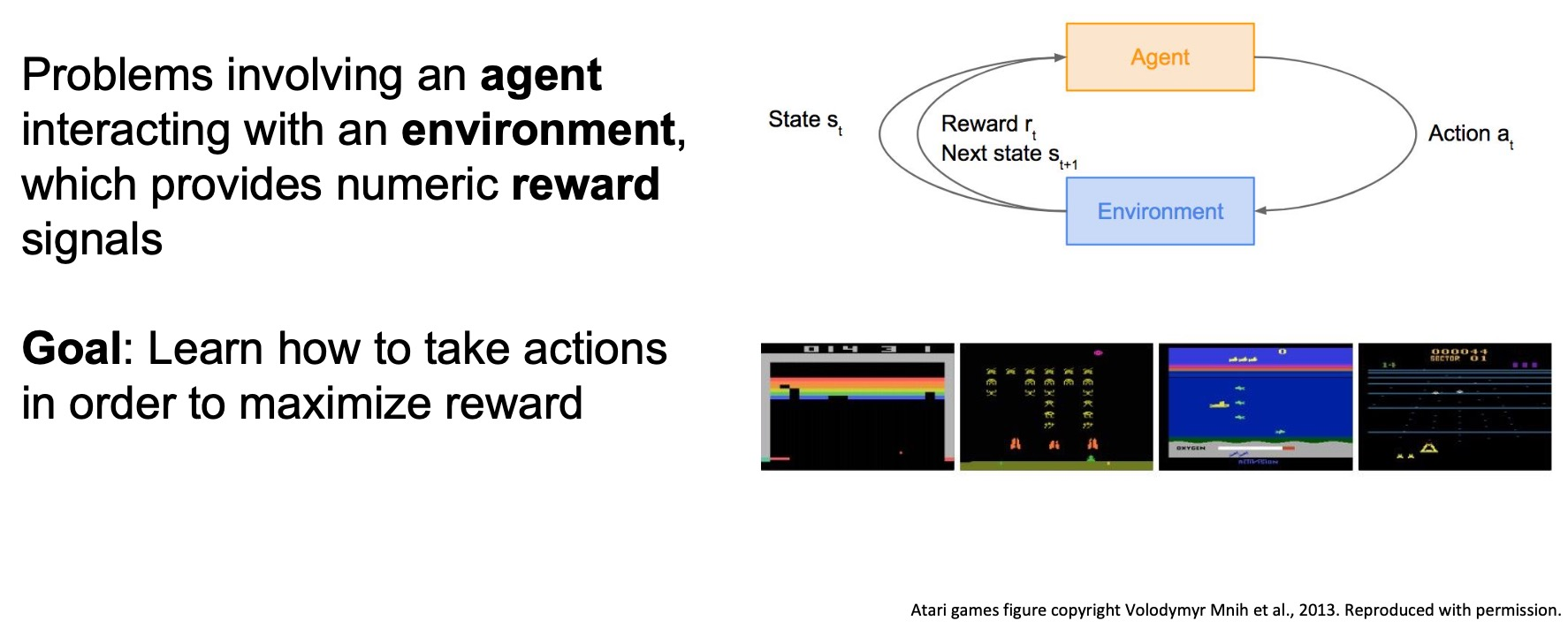• In Reinforcement Learning (RL), we have an agent that can take actions in an environment and receive rewards for its actions. The goal is to learn how to take actions in a way that can maximizes the rewards that the agent sees.
• At a high level, this will be different from what you’ve seen before for a couple of reasons:
• There’s indirect supervision happening through this reward that the agent obtains
• The agent is actually responsible for collecting its own data so we’re not going to provide it data explicitly – it has to choose what data it encounters.
• The problem is sequential in nature.

## What is Reinforcement Learning?

• In the RL setup, we have an agent and an environment and the agent is interacting with the environment.
• The figure below illustrates an episode of interaction between the agent and the environment:
• The environment gives the agent a state $$s_t$$ for observation.
• The agent takes an action $$a_t$$.
• The environment responds to this action by giving the agent a reward $$r_t$$.
• The environment transitions to the next state $$s_{t+1}$$.
• This process repeats until the environment reaches a terminal state/goal which ends the episode of interaction.
• RL problems are characterized by 6 parameters:
• Objective
• Agent
• Environment
• State
• Action
• Reward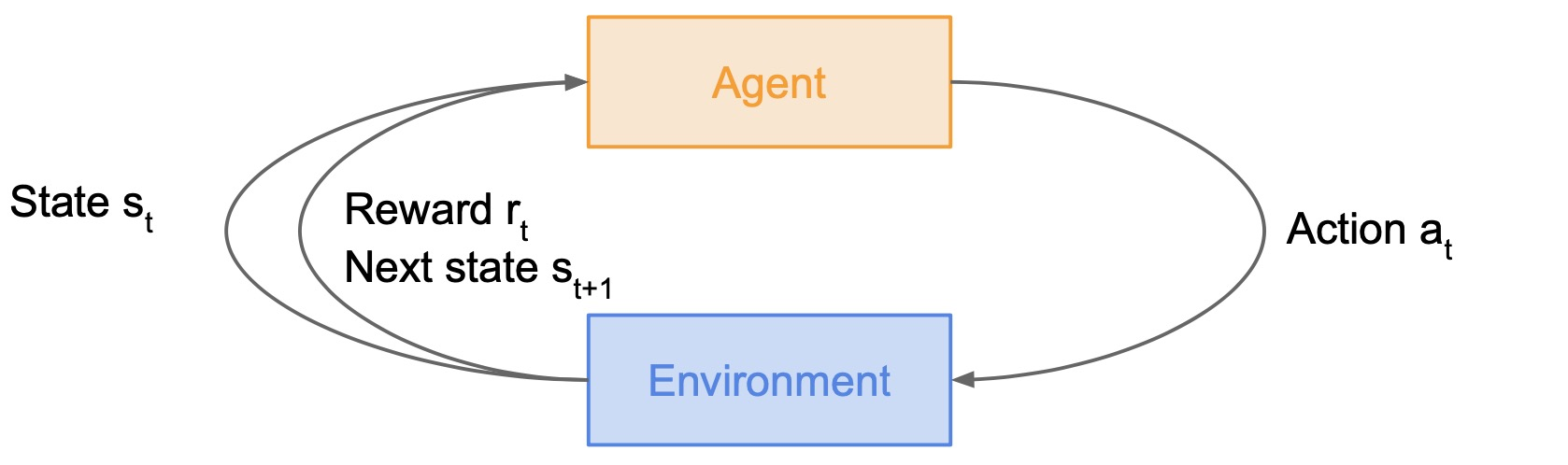### Cart-Pole Problem

• Here’s a Cart-Pole problem where the objective is to for the agent to balance a pole on top of a moveable cart.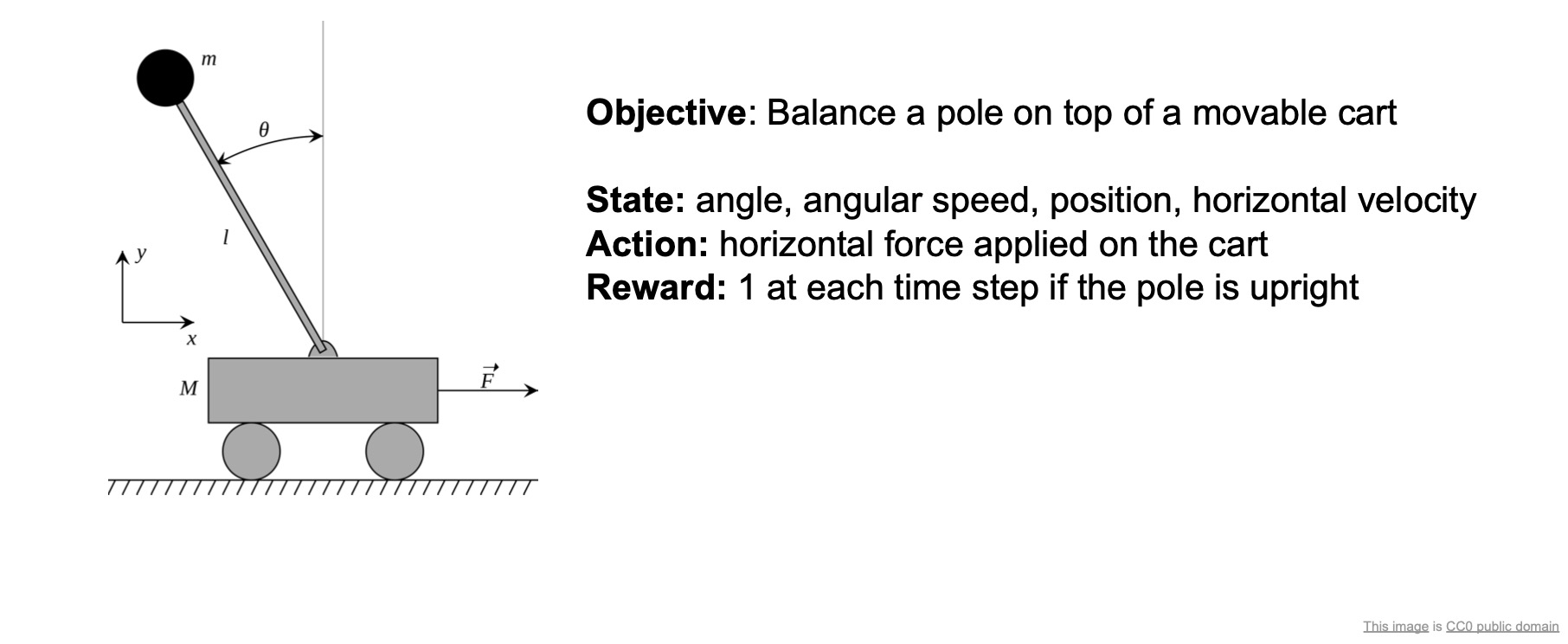• The state can include things like the angle between the pole and the vertical, the angular speed of the pole, the position and horizontal velocity of the cart – these are all decision variables that you might need to have in order to make good decisions on what to do next.
• The action space includes the action of appplying a certain amount of horizontal force on the cart. This is what the agent has to decide at every time step – how much force should I apply to the cart?
• The reward is 1 for each time step if the pole is upright. So, maximizing this reward is going to ensure that the agent learns to keep the pole upright.

### Robot Locomotion

• Another example is robot locomotion, as shown below.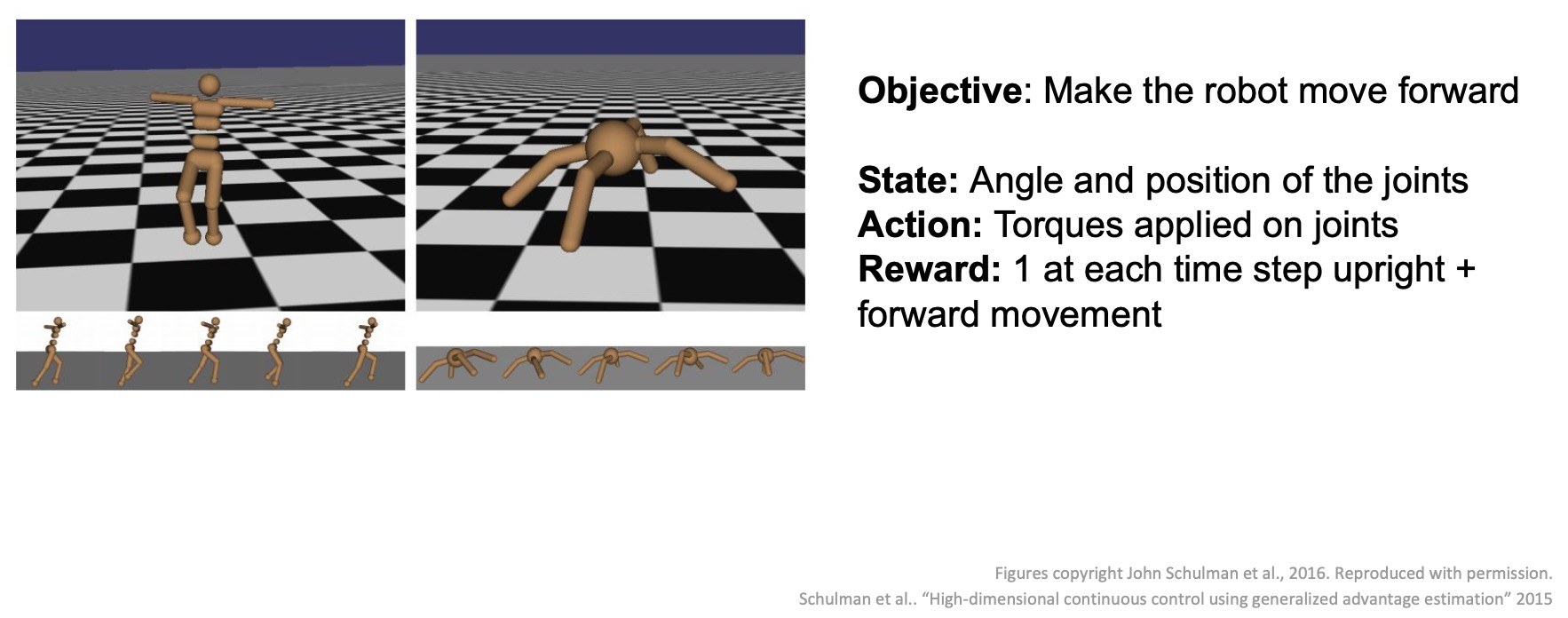• There’s a humanoid robot and an ant-robot model shown here in the above diagram.
• The objective is to make the robot move forward.
• The states might be the angles and positions of each of the joints.
• The action would be the torque to apply to each joint in order to move the robot.
• The reward might be 1 for every time step the robot is upright and for every time step where he actually gets some forward movement.

### Games

• Games are also a big class of problems that can be formulated with RL.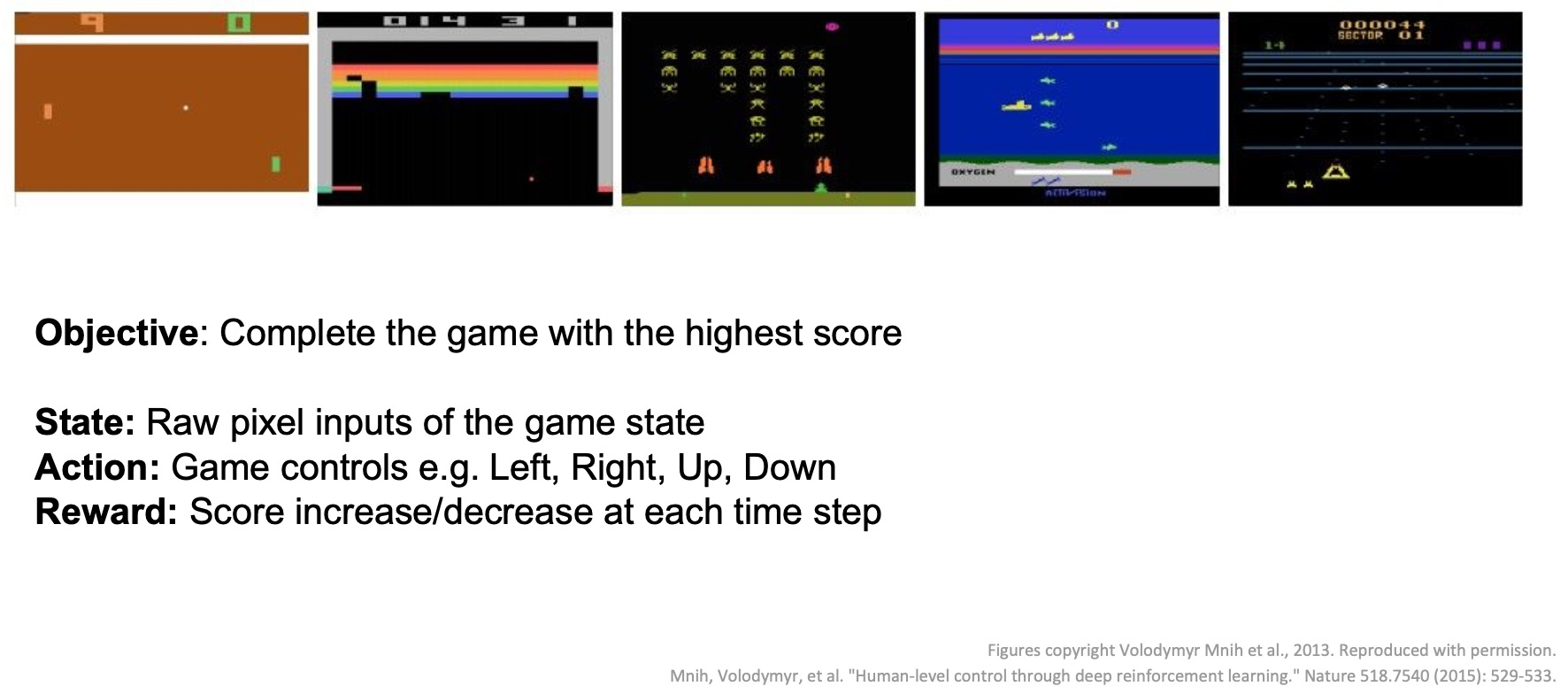• For e.g., we have Atari games which are a well-known success of deep RL.
• The agent is a player trying to play these games using visual inputs and game controls.
• The objective is to maximize the score or play the game as well as you can.
• In 2016, a huge milestone in this field was reached when DeepMind’s AlphaGo beat Lee Sedol, the best Go player in the history of the game.
• Playing the game of Go was formulated as a reinforcement learning problem.
• The state here was basically the position of all the pieces.
• The action was where to put the next piece down.
• The reward was 1 if you win the game or 0 otherwise.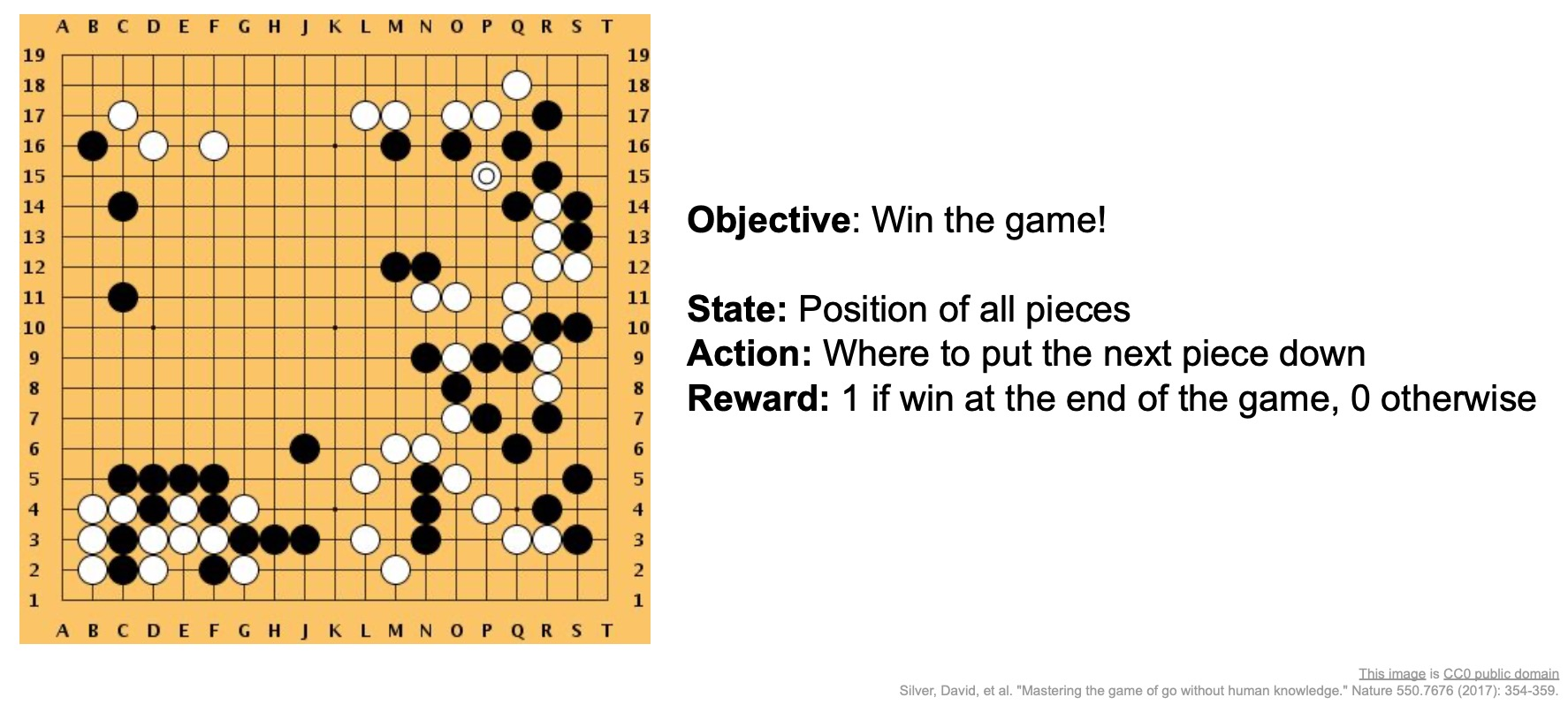## Markov Decision Processes (MDPs)

• MDPs help formalize the RL problem, which is the sequential interaction between the agent and the environment.
• Shown below is a mathematical formulation of the RL problem. MDPs satisfy the Markov property, a key property which says that the current environment state completely summarizes everything I need to know about the world and how it can change. In essence, that means that by only looking at the current state of the world, I’m able to make my decision in such a way that I can perform well at the task at hand.
• MDPs are defined by a tuple of objects: $$(S,\,A,\,R,\,P,\,\gamma)$$
• where,
• $$S$$ is the state space.
• $$A$$ is the action space.
• $$R$$ is the reward function or distribution, which is conditioned on state action pairs.
• $$P$$ is the transition probability, which tells us how does the world transition from one state to the next conditioned on the current state and an action that the agent takes.
• $$\gamma$$ is the discount factor, which tells us how much we value rewards now vs. later.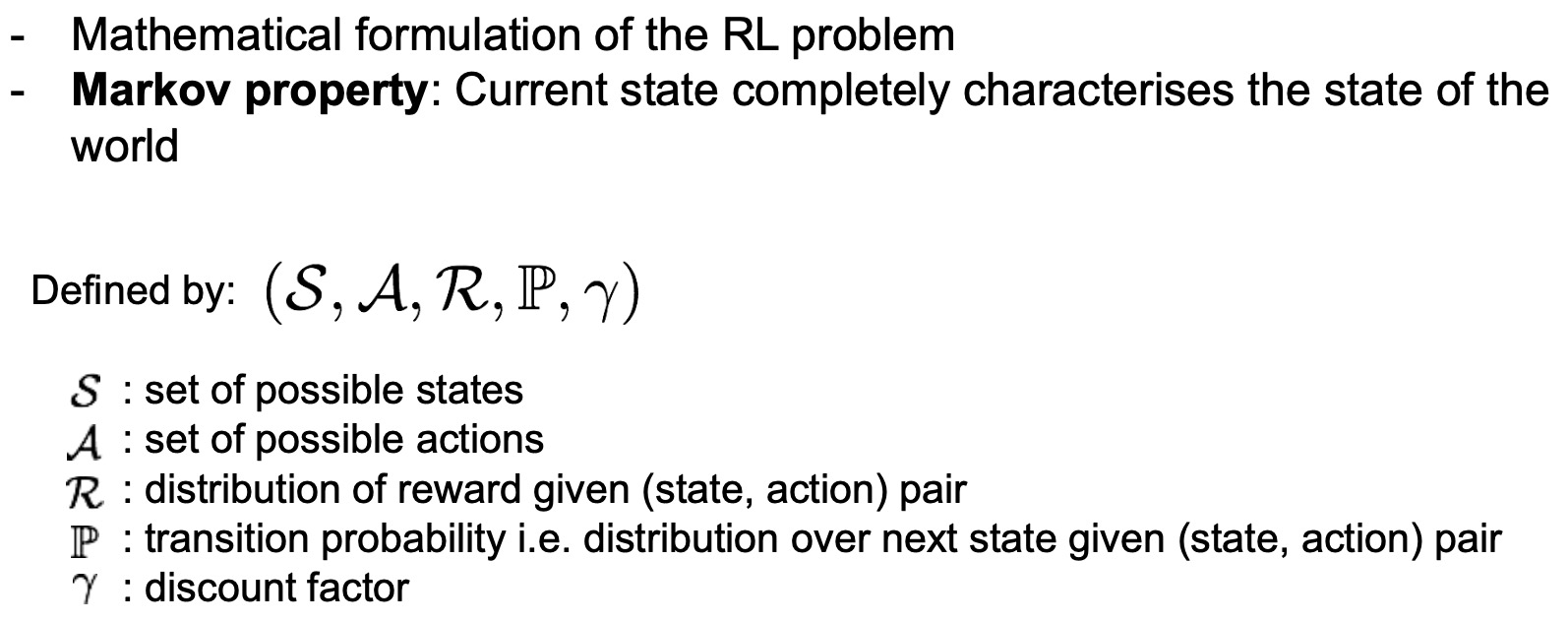• Here’s how an episode of interaction works between the agent and environment:
• At time step $$t\,=\,0$$, we begin a new episode of interaction where the environment samples an initial state $$s_0\,\sim\,p(s_0)$$ and places the agent in that state in the world.
• Next, we repeat the following:
• The agent selects an action $$a_t$$.
• The environment then takes that action and responds with both our reward $$r_t$$ and the next state $$s_{t+1}$$ according to their world and transition distributions.
• The agent receives the reward $$r_t$$ and the next state $$s_{t+1}$$, and then selects the next action $$a_{t+1}$$.
• This loop continues until the end of the episode.
• Next, let’s talk about an agent’s decision-making process.
• Policy:
• A policy is a function the maps $$states\,\rightarrow\,actions$$ or more generally a distribution of actions conditioned on states.
• It’s basically a set of rules that tell the agent what to do in every conceivable situation.
• Note that some decision-making rules are going to be better in terms of maximizing your rewards than others.
• Objective:
• To find the best policy $$\pi^*$$ or in other words, the best decision-making rule, that maximizes the cumulative discounted reward $$\sum\limits_{t\geq0} \gamma^t r_t$$.
• The discount factor $$\gamma$$ basically tells us how much do we care about rewards later vs. now.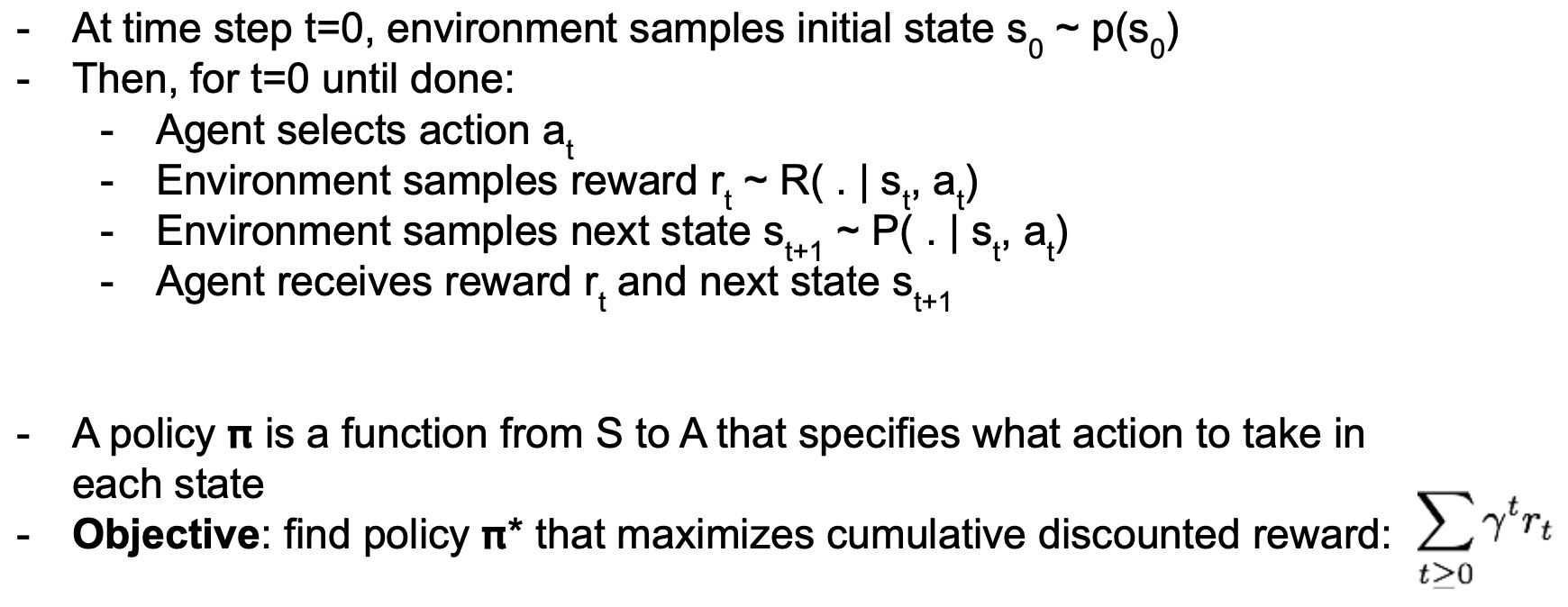#### Horizon of the Problem

• Does the horizon of the problem matter? Yes, it does!
• The horizon of the problem is defined by the number of steps the agent gets to interacts with the environment.
• When we talk about an MDP, a reward is offered at every single time step to the agent, but the agent has a certain horizon to play in the environment (characterized by the dicount $$\gamma$$) with the ultimate overarching goal of trying to maximize the sum of rewards.
• So the horizon definitely does matter. This has been one of the ongoing research questions in reinforcement learning which is how hard are longer horizon problems vs. shorter horizon problems.
• In the limiting case, if we just have to make a decision once and then we’re done with the episode, which seems intuitively a little bit easier, than having to make a long structured sequence of actions in order to achieve a task.
• In the theory of RL, the horizon of the problem does play an important role in both the definition and the difficulty of the problem.

#### The Problem of Spare Rewards

• In some cases, a reward can be sparse in a problem because we only know how to check success vs. failure but we don’t really know how to write down our reward function that will enable good behavior, i.e., which gives more dense supervision along the way.
• This is actually a classic problem especially in Robotics because sometimes we might know how to tell whether a task was successful or not, for e.g., if a robot has successfully lifted an object but knowing what type of reward function would guide the agent through this behavior isn’t straightforward. This can be hard because the agent needs to explore the action space and if it’s getting no rewards for the longest period of time until it accidentally lifts the object, it’s going to be a very hard problem to solve.
• Some fixes for this:
• Imitation learning: Learn the reward function from demonstrations of the task.

### Simple MDP: Grid World

• Shown below is a simple grid world example of an MDP.
• RL problem characteristics:
• The states are a discrete set of cells.
• The actions are to move right left up or down in this grid world.
• The reward function is that we get a negative reward for each transition (which implies, that we should be using the least number of steps to get to our terminal state).
• The goal (terminal state) is to reach one of these gray states as fast as possible.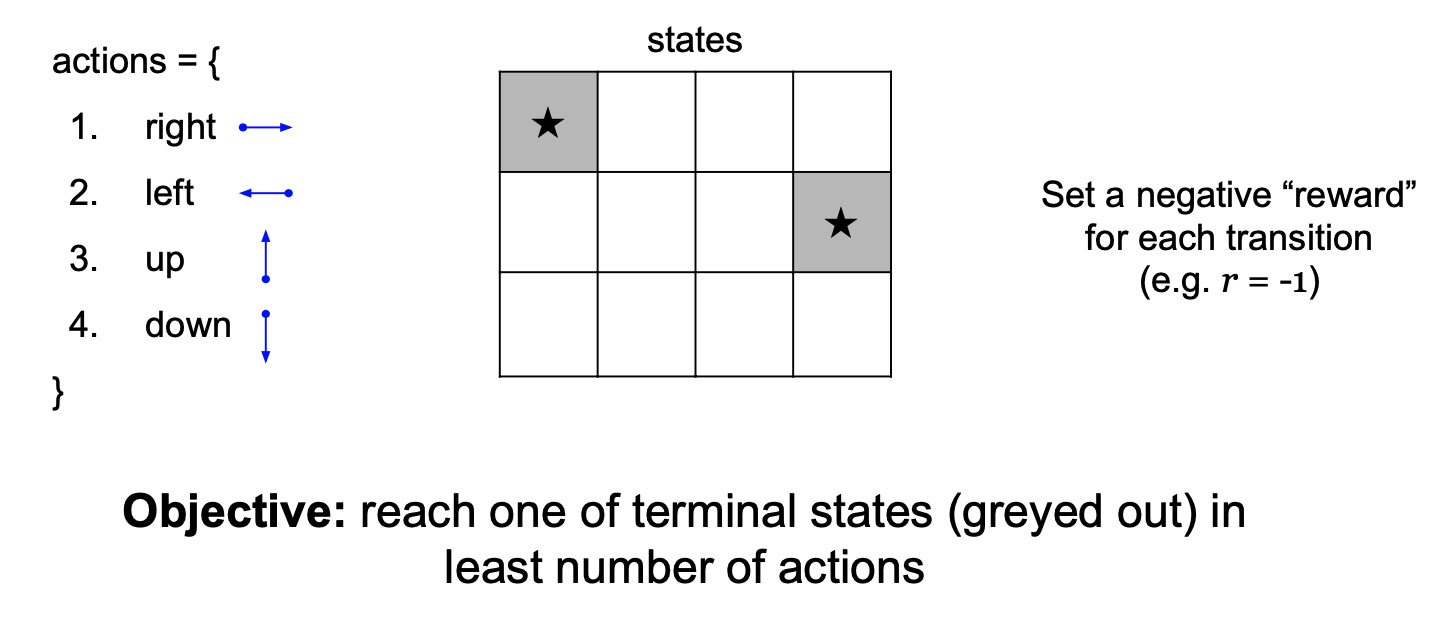### Simple MDP: Types of Policies

• We’re going to compare two different policies or decision-making rules here.
• Random policy: simply sample a random direction in every single grid cell at any given time step $$t$$.
• Optimal policy: tells us what are the possible actions that can be taken in every single grid cell such that we’ll need minimum number of time steps to reach the goal.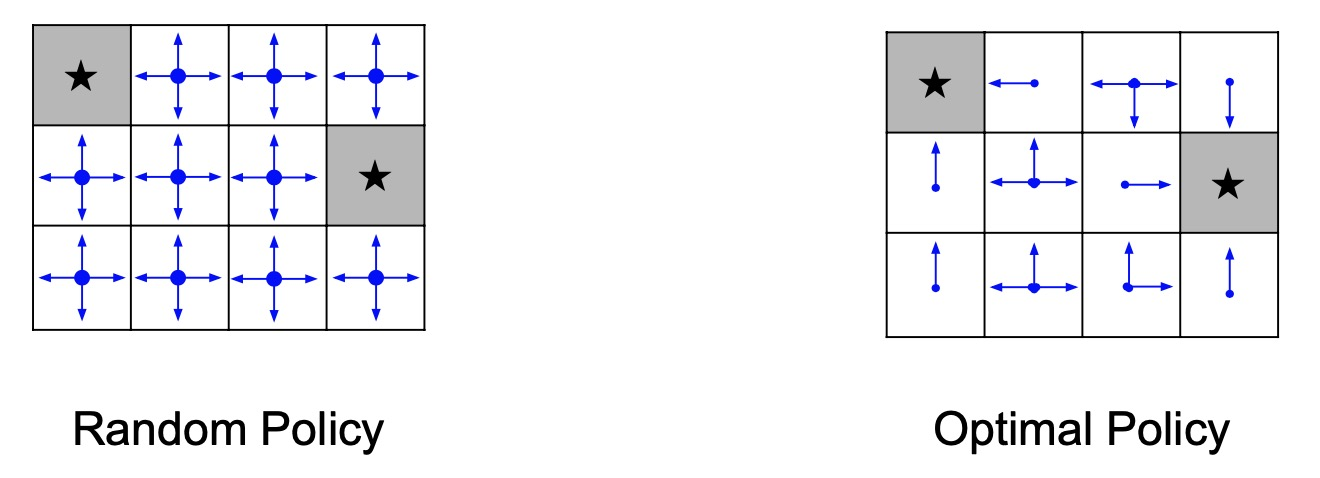### Simple MDP: Finding the Optimal Policy

• We’re looking to find the optimal policy $$\pi^*$$ that maximizes the sum of (discounted) rewards.
• This is going to be a decision-making rule that essentially tells us what to do in each grid cell.
• There can be a lot of randomness in the world with environmental aspects such as the initial state, the transitions, etc.
• Thus, the question arises: how can we characterize the performance of our policies given all these sources of randomness?
• Answer: We’ll maximize the expected some of the rewards.
• Formally, this is the RL problem (shown below). Our job is to find the best policy that maximizes the expected sum of rewards where we have randomness in the following:
• Start state
• Actions we choose if our policy as a distribution instead of a deterministic mapping
• How the world responds to our actions through the transition distribution.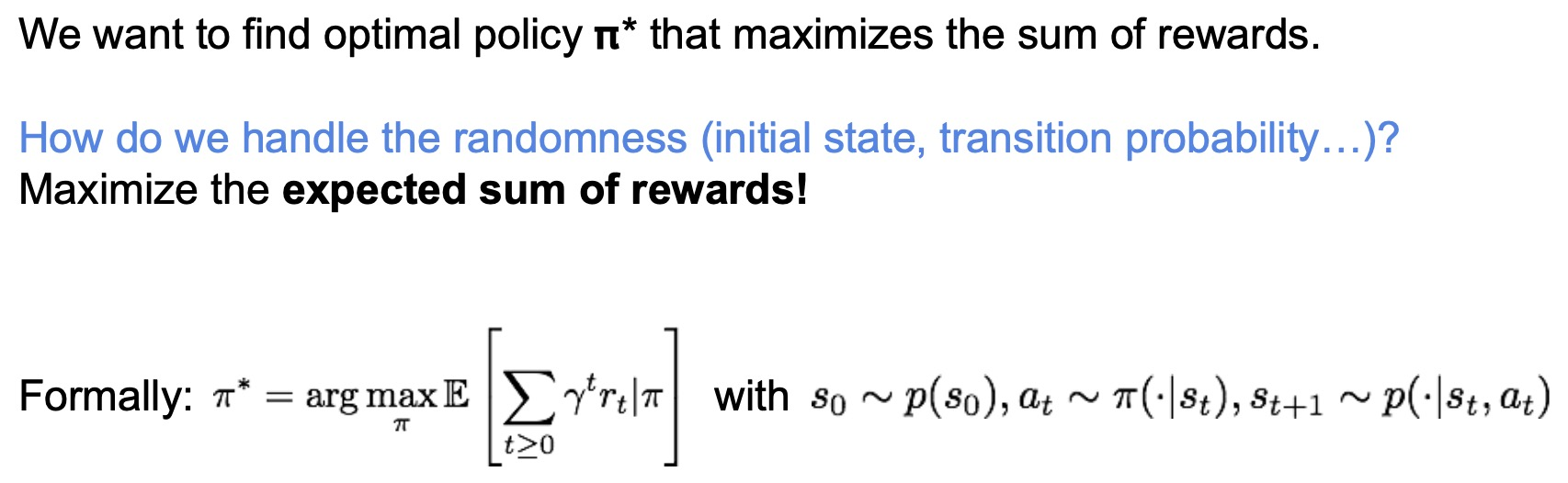• Before we talk about how to find the optimal policy, lets look into metrics that enable us to pick the best policy, namely the value function and Q function.

### Value Function

• The value function of a state $$s$$ is the average sum of rewards, i.e., the average return, that you can get if you start at that state and follow the policy (or the decision making rule) $$\pi$$.

• For a given policy (or decision-making rule), we sample many episodes of interaction starting from that start state. Next, we sum up all the rewards and average it out.
• Why is this useful?
• A value function offers an objective metric to compare one policy vs. another. Compare the value functions of two policies at a given state $$s$$ can help us find the better policy, since it will have a higher value function at that state $$s$$.

### Q Function / Q-Value Function / State Action Value Function

• A Q value function (or state action value function) is very similar to the value function except that we can take a different action at the first time step.
• Why is this useful?
• Sometimes we want to consider what would happen if we deviate from our decision making rule for the first time step $$t\,=\,0$$, by evaluating the impact of choosing a different action $$a$$.
• Intuitively, if deviating from $$\pi$$ by choosing a different action $$a$$ achieves higher value, then $$\pi$$ can’t be optimal since there’s actually a better strategy that exists.

## Q-Learning

• Q-Learning and Policy Gradients are the two major classes of RL algorithms.
• The optimal Q-value function $$Q^*$$ is given by the highest expected some rewards we can achieve in a given state $$s$$, after following a given action $$a$$.
• In other words, we’re looking to find the best policy/decision-making rule after a given action $$a$$ that maximizes sum of the rewards with $$Q^*$$ as the average return.
• Bellman equation
• One of the most fundamental ideas in RL the bellman equation.
• Given an optimal Q-value function $$Q*$$ which is the best you can do in a given $$(state, action)$$ pair, at the next time step you would better continue doing the same thing.
• In other words, assume you take action $$a$$ in state $$s$$. When you end up in the next state $$s’$$, the best thing for you to do is to select the action that maximizes my value which implies that we should maximize the Q function over actions in the next state.
• As always, we use an expectation because we’re going to have randomness over what state we’re going to end up in after taking that action $$a$$ in the original state.
• If $$Q^*$$ is the best you can do for any action, then the best policy is just going to take a maximum over actions at the current state. So the optimal policy $$\pi^*$$ is just the best action that maximizes $$Q^*$$.
• One of the big advantages of using value functions is that they remove the temporal aspect of the problem by summarizing everything that’s going to happen later so you can reduce it to a one step decision-making, which is simply to look at all your actions and pick the best one according to your Q function.

### Solve for the optimal policy

• Given the bellman equation, how do we solve for the optimal policy?
• one x one algorithm is called value iteration and it uses the bellman equation as an iterative update so here’s what we do
• i initialize my current Q values for every state in action at random and then I’ll plug these Q values into the right-hand side of the bellman equation and use it to extract a new set of Q values that’s the left-hand side so this is just an iterative update and notice that when I reach a fixed point which means my current Q values don’t change after an iteration that means the left-hand side equals the right-hand side the Q funk u function all my Q values will satisfy this equation um that means that the fixed point of this update rule is the as a bellman equation and thus it must be optimal so now the natural question is can I be sure to always reach a fixed point by iteratively applying this update there’s a remarkable fact the bellman update is what’s known as a contraction mapping what this means is if I do this update again and again and again I’m guaranteed to converge feet to the fixed point which is also the optimal Q function Q star so that’s exactly what value iteration does what seems reasonable but what’s the problem with this it’s not scalable we saw the grid world earlier what if we had a huge grid lots of states and lots of actions possible per state or we had a continuous space instead of a discrete one like image observations in Atari we can’t store a table of Q values for every single state and every single action so what’s the potential solution to this well why not just throw a function approximator edit let’s learn a mapping between state action pairs and give values instead of storing every single pair of inputs and outputs in a table.
• Neural Networks can serve as effective function approximators, so let’s try that so we’re going to use a function approximator and this is what’s going to lead us to something called deep Q learning, a common part of modern era artificial intelligence.
• If the function approximator is the function approximator is parameterize by a set of weights theta so these conduces you can think of this as the weights of our neural network and by varying these weights of my neural network we’re going to obtain different cue functions and the cue learning problem is now going to be find me the best set of neural network weights the best theta that’s going to get closest to the optimal Q function in this Q start and consequently produce the best behavior for my agent so how can we learn that
• In RL almost everything is about the bellman equation so let’s start from there what we’re gonna do is we want to find a cute Q function that satisfies the bellman equation but and we would like to do something like value iteration but we don’t have a way to do an exact update we don’t know how to do this exact update in closed form but we do know how to do that propagation for neural networks so why don’t we just put a penalty on how much the current Q function D leads from the bellman equation I can look at the left-hand side and right-hand side of the bellman equation and I can say um how much does the current Q function how much error doesn’t achieve between the left hand right-hand side and I put an l2 loss on that so you can see here is y I is given by the right-hand side of the bellman equation it says with my current Q function what does the right-hand side look like and then the left-hand side is just my incurrent q function at the given state in action I put up a square L to us and I minimize that so that’s exactly what we’re gonna do and this is what is known as DQ learning I’m going to use a function approximator for my Q network and I’m going to approximately try and solve the bellman equation through a value iteration by keep by minimizing this l2 loss

• Reinforcement learning problems are involving an agent interacting with an environment, which provides numeric reward signals.
• Steps are:
• Environment –> State s[t] –> Agent –> Action a[t] –> Environment –> Reward r[t] + Next state s[t+1] –> Agent –> and so on..
• Our goal is learn how to take actions in order to maximize reward.
• An example is Robot Locomotion:
• Objective: Make the robot move forward
• State: Angle and position of the joints
• Action: Torques applied on joints
• 1 at each time step upright + forward movement
• Another example is Atari Games:
• Deep learning has a good state of art in this problem.
• Objective: Complete the game with the highest score.
• State: Raw pixel inputs of the game state.
• Action: Game controls e.g. Left, Right, Up, Down
• Reward: Score increase/decrease at each time step
• Go game is another example which AlphaGo team won in the last year (2016) was a big achievement for AI and deep learning because the problem was so hard.
• We can mathematically formulate the RL (reinforcement learning) by using **Markov Decision Process**
• Markov Decision Process
• Defined by (S, A, R, P, Y) where:
• S: set of possible states.
• A: set of possible actions
• R: distribution of reward given (state, action) pair
• P: transition probability i.e. distribution over next state given (state, action) pair
• Y: discount factor # How much we value rewards coming up soon verses later on.
• Algorithm:
• At time step t=0, environment samples initial state s
• Then, for t=0 until done:
• Agent selects action a[t]
• Environment samples reward from R with (s[t], a[t])
• Environment samples next state from P with (s[t], a[t])
• Agent receives reward r[t] and next state s[t+1]
• A policy pi is a function from S to A that specifies what action to take in each state.
• Objective: find policy pi* that maximizes cumulative discounted reward: Sum(Y^t * r[t], t>0)
• For example:
•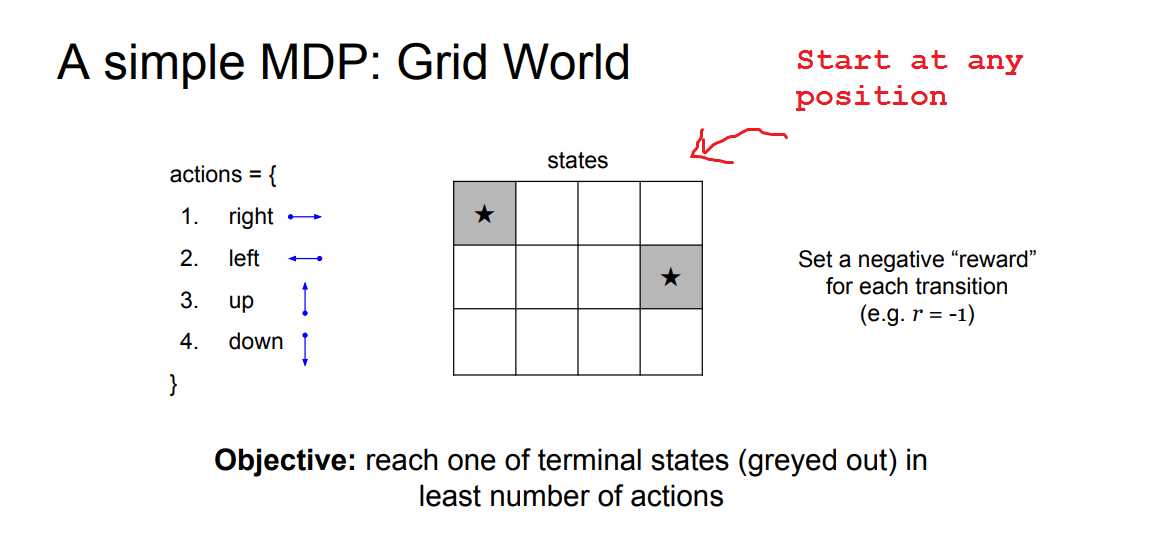• Solution would be:
•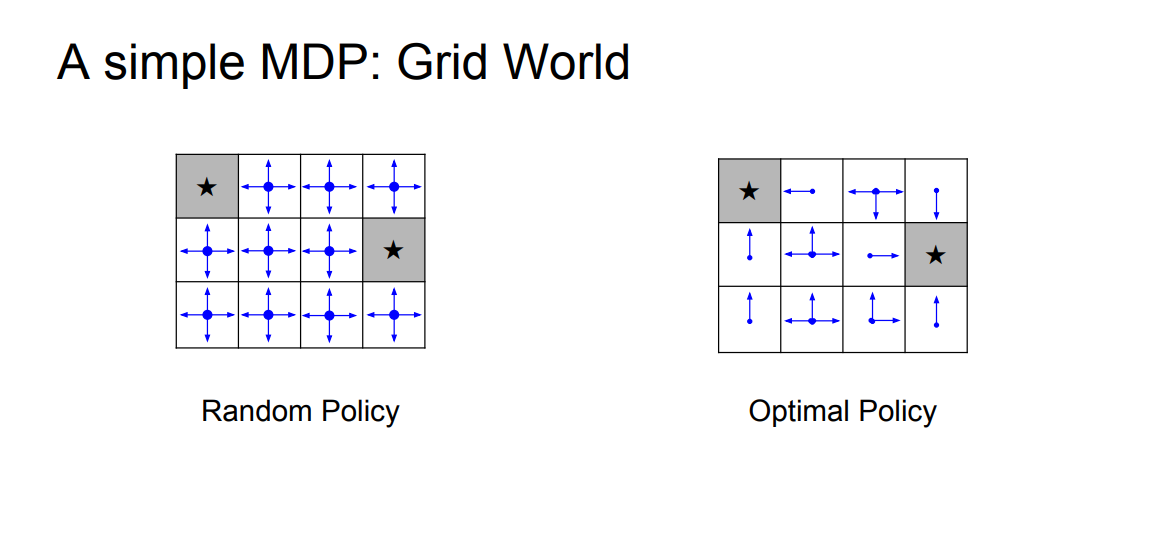• The value function at state s, is the expected cumulative reward from following the policy from state s:
• V[pi](s) = Sum(Y^t * r[t], t>0) given s0 = s, pi
• The Q-value function at state s and action a, is the expected cumulative reward from taking action a in state s and then following the policy:
• Q[pi](s,a) = Sum(Y^t * r[t], t>0) given s0 = s,a0 = a, pi
• The optimal Q-value function Q* is the maximum expected cumulative reward achievable from a given (state, action) pair:
• Q*[s,a] = Max(for all of pi on (Sum(Y^t * r[t], t>0) given s0 = s,a0 = a, pi))
• Bellman equation
• Important thing is RL.
• Given any state action pair (s,a) the value of this pair is going to be the reward that you are going to get r plus the value of the state that you end in.
• Q*[s,a] = r + Y * max Q*(s',a') given s,a # Hint there is no policy in the equation
• The optimal policy pi* corresponds to taking the best action in any state as specified by Q*
• We can get the optimal policy using the value iteration algorithm that uses the Bellman equation as an iterative update
•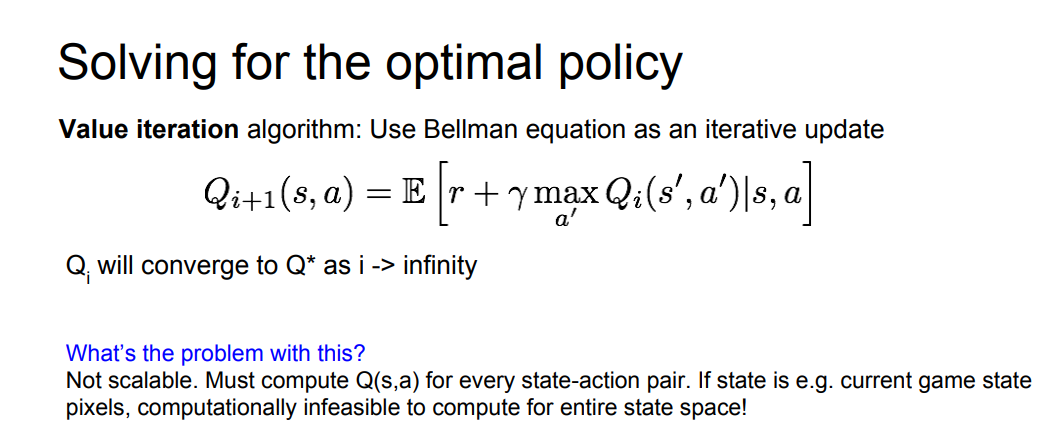• Due to the huge space dimensions in real world applications we will use a function approximator to estimate Q(s,a). E.g. a neural network! this is called Q-learning
• Any time we have a complex function that we cannot represent we use Neural networks!
• Q-learning
• The first deep learning algorithm that solves the RL.
• Use a function approximator to estimate the action-value function
• If the function approximator is a deep neural network => deep q-learning
• The loss function:
•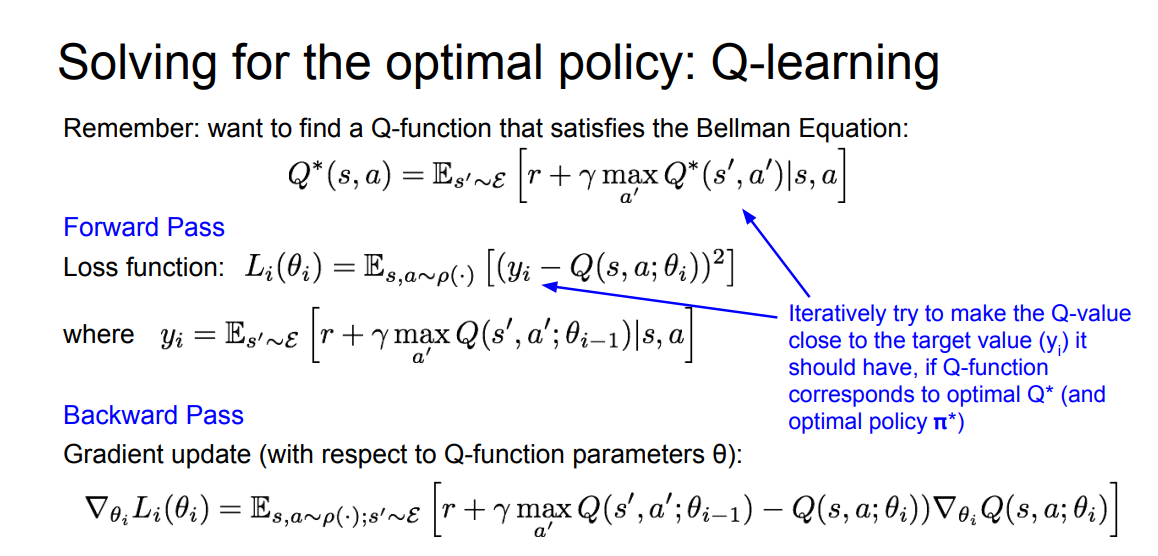• Now lets consider the “Playing Atari Games” problem:
• Our total reward are usually the reward we are seeing in the top of the screen.
• Q-network Architecture:
•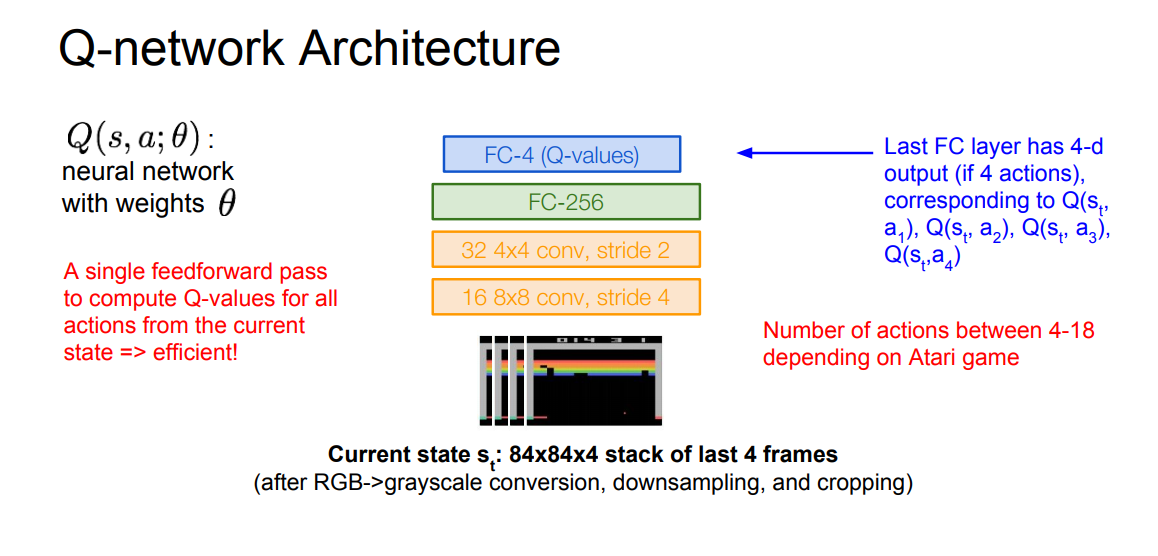• Learning from batches of consecutive samples is a problem. If we recorded a training data and set the NN to work with it, if the data aren’t enough we will go to a high bias error. so we should use “experience replay” instead of consecutive samples where the NN will try the game again and again until it masters it.
• Continually update a replay memory table of transitions (s[t] , a[t] , r[t] , s[t+1]) as game (experience) episodes are played.
• Train Q-network on random minibatches of transitions from the replay memory, instead of consecutive samples.
• The full algorithm:
•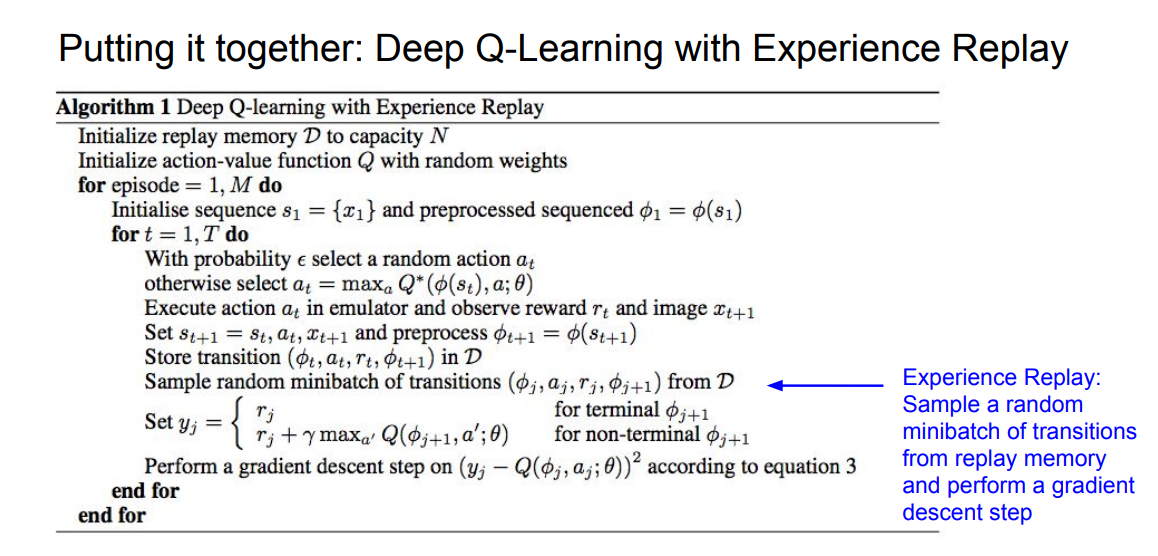• A video that demonstrate the algorithm on Atari game can be found here: “https://www.youtube.com/watch?v=V1eYniJ0Rnk”
• The second deep learning algorithm that solves the RL.
• The problem with Q-function is that the Q-function can be very complicated.
• Example: a robot grasping an object has a very high-dimensional state.
• But the policy can be much simpler: just close your hand.
• Can we learn a policy directly, e.g. finding the best policy from a collection of policies?
• Policy Gradients equations:
•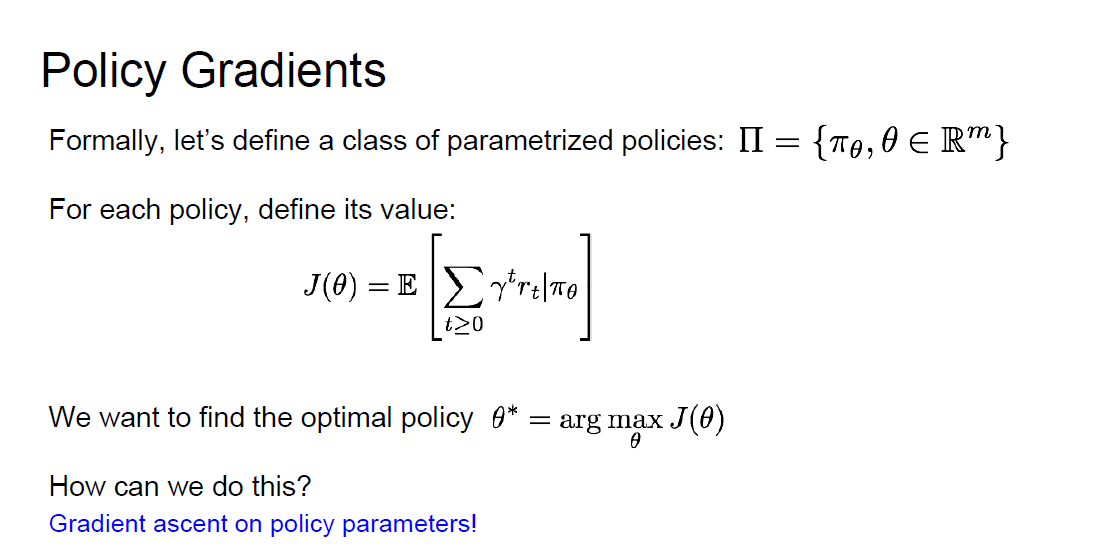• Converges to a local minima of J(ceta), often good enough!
• REINFORCE algorithm is the algorithm that will get/predict us the best policy
• Equation and intuition of the Reinforce algorithm:
•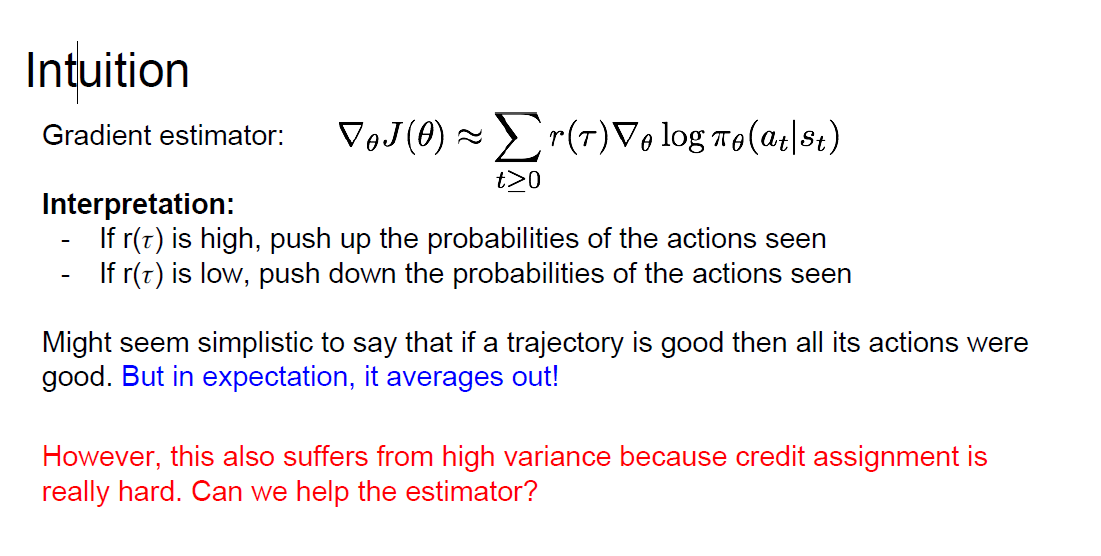• the problem was high variance with this equation can we solve this?
• variance reduction is an active research area!
• Recurrent Attention Model (RAM) is an algorithm that are based on REINFORCE algorithm and is used for image classification problems:
• Take a sequence of “glimpses” selectively focusing on regions of the image, to predict class
• Inspiration from human perception and eye movements.
• Saves computational resources => scalability
• If an image with high resolution you can save a lot of computations
• Able to ignore clutter / irrelevant parts of image
• RAM is used now in a lot of tasks: including fine-grained image recognition, image captioning, and visual question-answering
• AlphaGo are using a mix of supervised learning and reinforcement learning, It also using policy gradients.
• A good course from Standford on deep reinforcement learning
• http://web.stanford.edu/class/cs234/index.html
• A good course on deep reinforcement learning (2017)
• http://rll.berkeley.edu/deeprlcourse/
• A good article
• https://www.kdnuggets.com/2017/09/5-ways-get-started-reinforcement-learning.html

Here are some (optional) links you may find interesting for further reading:

## Citation

If you found our work useful, please cite it as:

@article{Chadha2020DistilledDeepReinforcementLearning,
title   = {Deep Reinforcement Learning},
author  = {Chadha, Aman},
journal = {Distilled Notes for Stanford CS231n: Convolutional Neural Networks for Visual Recognition},
year    = {2020",
note    = {\url{https://aman.ai}}
}